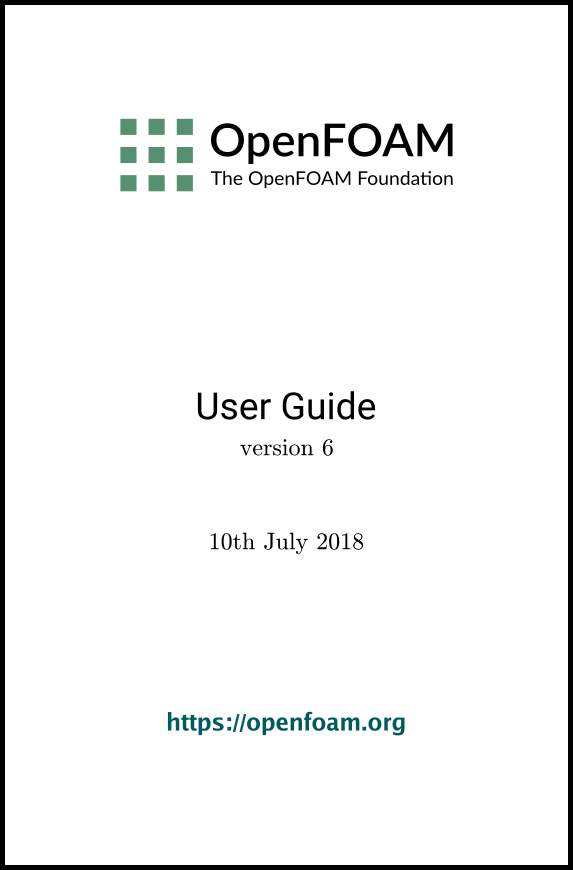## 7.1 Thermophysical models

Thermophysical models are concerned with energy, heat and physical properties. The thermophysicalProperties dictionary is read by any solver that uses the thermophysical model library. A thermophysical model is constructed in OpenFOAM as a pressure-temperature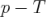system from which other properties are computed. There is one compulsory dictionary entry called thermoType which specifies the package of thermophysical modelling that is used in the simulation. OpenFOAM includes a large set of pre-compiled combinations of modelling, built within the code using C++ templates. This coding approach assembles thermophysical modelling packages beginning with the equation of state and then adding more layers of thermophysical modelling that derive properties from the previous layer(s). The keyword entries in thermoType reflects the multiple layers of modelling and the underlying framework in which they combined. Below is an example entry for thermoType:

thermoType
{
type            hePsiThermo;
mixture         pureMixture;
transport       const;
thermo          hConst;
equationOfState perfectGas;
specie          specie;
energy          sensibleEnthalpy;
}

The keyword entries specify the choice of thermophysical models, e.g. constant transport (constant viscosity, thermal diffusion), Perfect Gas equationOfState, etc. In addition there is a keyword entry named energy that allows the user to specify the form of energy to be used in the solution and thermodynamics. The following sections explains the entries and options in the thermoType package.

### 7.1.1 Thermophysical and mixture models

Each solver that uses thermophysical modelling constructs an object of a specific thermophysical model class. The model classes are listed below.

psiThermo
Thermophysical model for fixed composition, based on compressibility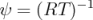, where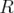is Gas Constant and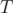is temperature. The solvers that construct psiThermo include the compressible family of solvers (sonicFoam, simpleFoam, etc., excluding rhoPorousSimpleFoam) and uncoupledKinematicParcelFoam and coldEngineFoam.
rhoThermo
Thermophysical model for fixed composition, based on density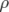. The solvers that construct rhoThermo include the heatTransfer family of solvers (buoyantSimpleFoam, CHT solvers, etc., excluding Boussinesq solvers) and rhoPorousSimpleFoam, twoPhaseEulerFoam and thermoFoam.
psiReactionThermo
Thermophysical model for reacting mixture, based on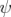. The solvers that construct psiReactionThermo include many of the combustion solvers, e.g.sprayFoam, chemFoam, fireFoam and reactingFoam, and some lagrangian solvers, e.g. coalChemistryFoam and reactingParcelFilmFoam.
psiuReactionThermo
Thermophysical model for combustion, based on compressibility of unburnt gas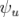. The solvers that construct psiuReactionThermo include combustion solvers that model combustion based on laminar flame speed and regress variable, e.g.XiFoam, PDRFoam and engineFoam.
rhoReactionThermo
Thermophysical model for reacting mixture, based on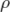. The solvers that construct rhoReactionThermo include some combustion solvers, e.g.rhoReactingFoam, rhoReactingBuoyantFoam, and some lagrangian solvers, e.g. reactingParcelFoam and simpleReactingParcelFoam.
multiphaseMixtureThermo
Thermophysical models for multiple phases. The solvers that construct multiphaseMixtureThermo include compressible multiphase interface-capturing solvers, e.g.compressibleInterFoam, and compressibleMultiphaseInterFoam.

Note: a new generalised fluidThermo model currently exists that allows the user to specify the thermophysical model through the type entry (described below) at run-time. Currently only the rhoSimpleFoam and rhoPimpleFoam solvers construct the generalised fluidThermo.

The type keyword specifies the underlying thermophysical model. Options are listed below.

• hePsiThermo: for solvers that construct psiThermo and psiReactionThermo.
• heRhoThermo: for solvers that construct rhoThermo, rhoReactionThermo and multiphaseMixtureThermo.
• heheuPsiThermo: for solvers that construct psiuReactionThermo.

The mixture specifies the mixture composition. The option typically used for thermophysical models without reactions is pureMixture, which represents a mixture with fixed composition. When pureMixture is specified, the thermophysical models coefficients are specified within a sub-dictionary called mixture.

For mixtures with variable composition, required by thermophysical models with reactions, the reactingMixture option is used. Species and reactions are listed in a chemistry file, specified by the foamChemistryFile keyword. The reactingMixture model then requires the thermophysical models coefficients to be specified for each specie within sub-dictionaries named after each specie, e.g. O2, N2.

For combustion based on laminar flame speed and regress variables, constituents are a set of mixtures, such as fuel, oxidant and burntProducts. The available mixture models for this combustion modelling are homogeneousMixture, inhomogeneousMixture and veryInhomogeneousMixture.

Other models for variable composition are egrMixture, multiComponentMixture and singleStepReactingMixture.

### 7.1.2 Transport model

The transport modelling concerns evaluating dynamic viscosity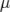, thermal conductivity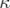and thermal diffusivity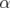(for internal energy and enthalpy equations). The current transport models are as follows:

const
assumes a constant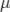and Prandtl number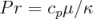which is simply specified by a two keywords, mu and Pr, respectively.
sutherland
calculates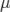as a function of temperature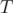from a Sutherland coefficient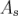and Sutherland temperature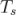, specified by keywords As and Ts;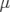is calculated according to: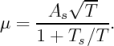(7.1)
polynomial
calculates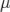and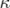as a function of temperature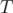from a polynomial of any order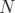, e.g.: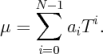(7.2)
logPolynomial
calculates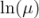and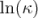as a function of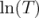from a polynomial of any order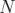; from which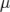,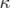are calculated by taking the exponential, e.g.: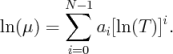(7.3)

### 7.1.3 Thermodynamic models

The thermodynamic models are concerned with evaluating the specific heat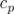from which other properties are derived. The current thermo models are as follows:

hConst
assumes a constant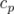and a heat of fusion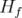which is simply specified by a two values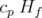, given by keywords Cp and Hf.
eConst
assumes a constant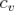and a heat of fusion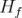which is simply specified by a two values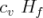, given by keywords Cv and Hf.
janaf
calculates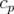as a function of temperature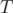from a set of coefficients taken from JANAF tables of thermodynamics. The ordered list of coefficients is given in Table 7.1. The function is valid between a lower and upper limit in temperature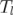and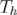respectively. Two sets of coefficients are specified, the first set for temperatures above a common temperature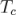(and below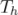), the second for temperatures below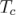(and above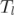). The function relating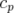to temperature is: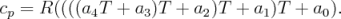(7.4)
In addition, there are constants of integration,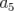and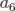, both at high and low temperature, used to evaluating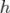andrespectively.
hPolynomial
calculates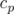as a function of temperature by a polynomial of any order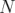: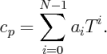(7.5)
The following case provides an example of its use: \$FOAM_TUTORIALS/lagrangian/porousExplicitSourceReactingParcelFoam/filter

 Description Entry Keyword Lower temperature limit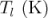Tlow Upper temperature limit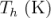Thigh Common temperature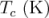Tcommon High temperature coefficients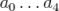highCpCoeffs (a0 a1 a2 a3 a4... High temperature enthalpy offset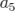a5... High temperature entropy offset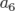a6) Low temperature coefficients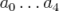lowCpCoeffs (a0 a1 a2 a3 a4... Low temperature enthalpy offset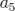a5... Low temperature entropy offseta6)

Table 7.1: JANAF thermodynamics coefficients.

### 7.1.4 Composition of each constituent

There is currently only one option for the specie model which specifies the composition of each constituent. That model is itself named specie, which is specified by the following entries.

• nMoles: number of moles of component. This entry is only used for combustion modelling based on regress variable with a homogeneous mixture of reactants; otherwise it is set to 1.
• molWeight in grams per mole of specie.

### 7.1.5 Equation of state

The following equations of state are available in the thermophysical modelling library.

rhoConst
Constant density: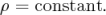(7.6)
perfectGas
Perfect gas: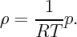(7.7)
incompressiblePerfectGas
Perfect gas for an incompressible fluid: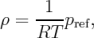(7.8)
where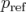is a reference pressure.
perfectFluid
Perfect fluid: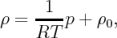(7.9)
where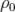is the density at.
linear
Linear equation of state: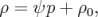(7.10)
where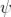is compressibility (not necessarily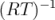).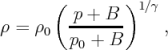(7.11)
where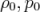are reference density and pressure respectively, and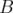is a model constant.
Boussinesq
Boussinesq approximation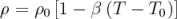(7.12)
where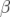is the coeffient of volumetric expansion and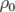is the reference density at reference temperature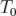.
PengRobinsonGas
Peng Robinson equation of state: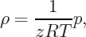(7.13)
where the complex function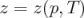can be referenced in the source code in PengRobinsonGasI.H, in the \$FOAM_SRC/thermophysicalModels/specie/equationOfState/ directory.
icoPolynomial
Incompressible, polynomial equation of state: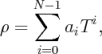(7.14)
where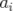are polynomial coefficients of any order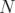.

### 7.1.6 Selection of energy variable

The user must specify the form of energy to be used in the solution, either internal energyand enthalpy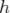, and in forms that include the heat of formation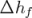or not. This choice is specified through the energy keyword.

We refer to absolute energy where heat of formation is included, and sensible energy where it is not. For example absolute enthalpy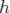is related to sensible enthalpy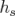by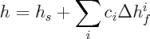(7.15)
where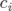and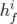are the molar fraction and heat of formation, respectively, of specie. In most cases, we use the sensible form of energy, for which it is easier to account for energy change due to reactions. Keyword entries for energy therefore include e.g. sensibleEnthalpy, sensibleInternalEnergy and absoluteEnthalpy.

### 7.1.7 Thermophysical property data

The basic thermophysical properties are specified for each species from input data. Data entries must contain the name of the specie as the keyword, e.g. O2, H2O, mixture, followed by sub-dictionaries of coefficients, including:

specie
containing i.e. number of moles, nMoles, of the specie, and molecular weight, molWeight in units of g/mol;
thermodynamics
containing coefficients for the chosen thermodynamic model (see below);
transport
containing coefficients for the chosen tranpsort model (see below).

The following is an example entry for a specie named fuel modelled using sutherland transport and janaf thermodynamics:

fuel
{
specie
{
nMoles       1;
molWeight    16.0428;
}
thermodynamics
{
Tlow         200;
Thigh        6000;
Tcommon      1000;
highCpCoeffs (1.63543 0.0100844 -3.36924e-06 5.34973e-10
-3.15528e-14 -10005.6 9.9937);
lowCpCoeffs  (5.14988 -0.013671 4.91801e-05 -4.84744e-08
1.66694e-11 -10246.6 -4.64132);
}
transport
{
As           1.67212e-06;
Ts           170.672;
}
}
The following is an example entry for a specie named air modelled using const transport and hConst thermodynamics:

air
{
specie
{
nMoles          1;
molWeight       28.96;
}
thermodynamics
{
Cp              1004.5;
Hf              2.544e+06;
}
transport
{
mu              1.8e-05;
Pr              0.7;
}
}
OpenFOAM v6 User Guide - 7.1 Thermophysical models